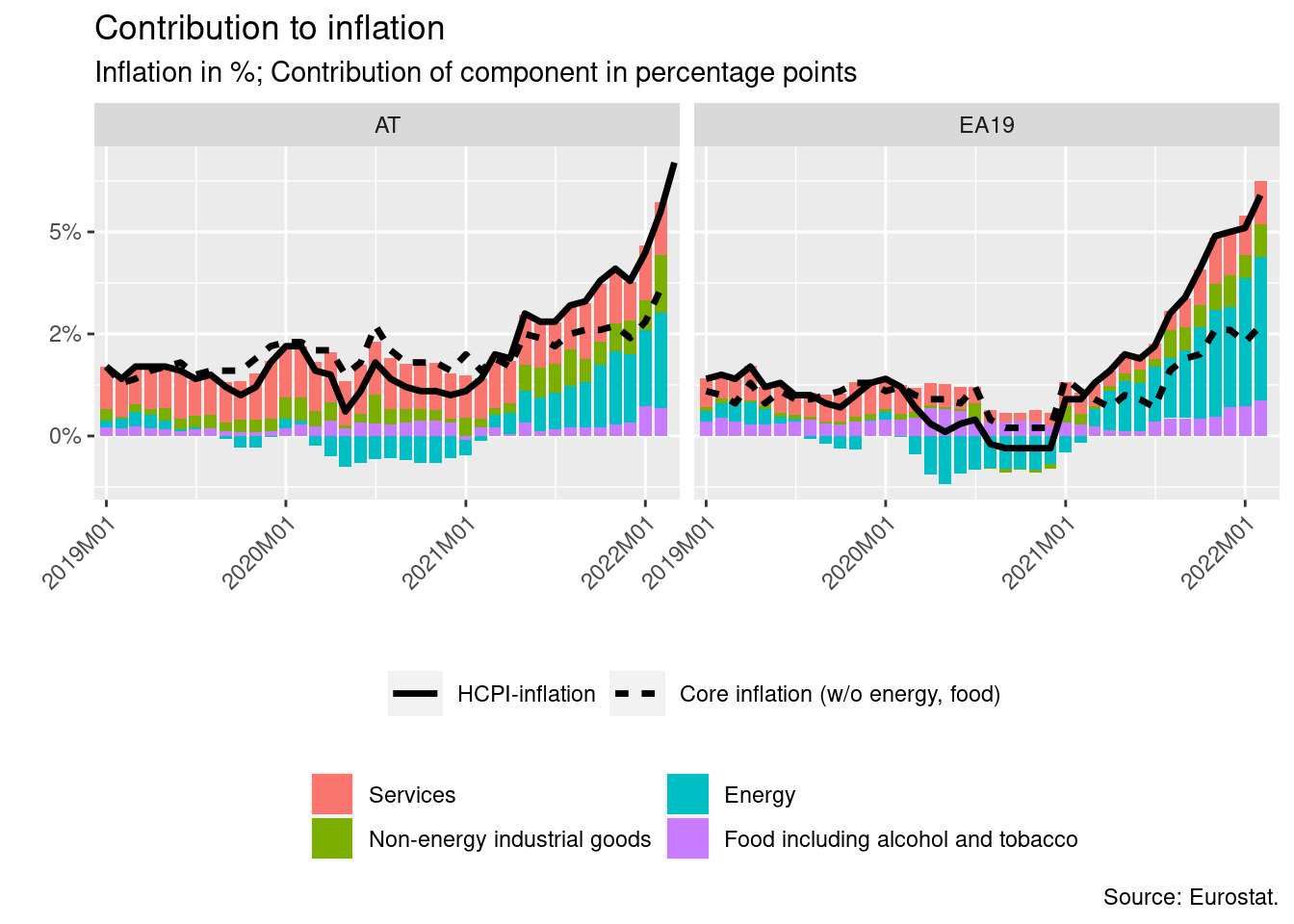# Decomposing Inflation for EA Countries

As a result of supply chain disruptions due to the Covid-19 pandemic and the recent war in the Ukraine inflation has reached unusually high levels during the past months. A first approach to analyse the evolution of inflation over time is to look at its main components: energy, food, services and industrial goods. To do this, I use data from Eurostat, which can be easily loaded into R using the eurostat package for countries of the European Union.

Before loading the data, define the set of countries, for which the decomposition should be done. The code below allows to compare the decompositions of inflation for multiple countries. Therefore, a vector can be provided. For this example, I use data on Austria and the euro area.

ctry <- c("AT", "EA19")

Define the start date:

start_date <- "2019-01-01"

# Load packages
library(dplyr)
library(eurostat)
library(ggplot2)

Download data on the weights of the individual components, which describe the relative importance of the respective component in overall inflation. They sum up to 1000.

# Weights
weights <- get_eurostat(id = "prc_hicp_inw",
filters = list(geo = ctry,
coicop = c("FOOD", "NRG", "IGD_NNRG", "SERV")))

weights <- weights %>%
# Generate year column for merge with monthly index-growth data
mutate(year = substring(time, 1, 4)) %>%
select(-time) %>%
rename(weight = values)

index <- get_eurostat(id = "prc_hicp_manr",
filters = list(geo = ctry,
coicop = c("CP00", "TOT_X_NRG_FOOD", "FOOD", "NRG", "IGD_NNRG", "SERV"))) %>%
filter(time >= start_date,
!is.na(values)) %>%
# Prepare percentage data for ggplot
mutate(values = values / 100)

Combine the series of component-specific growth rates with the weights.

comp <- index %>%
# Drop series, which are not used for decomposition
filter(!coicop %in% c("CP00", "TOT_X_NRG_FOOD")) %>%
# Gernate year column for merge with weights
mutate(year = substring(time, 1, 4)) %>%
# Merge with weights
left_join(weights, by = c("year", "geo", "coicop")) %>%
# Obtain values for composition
group_by(time, geo) %>%
mutate(weight = weight / sum(weight),
values = values * weight) %>%
ungroup() %>%
# Last formatting for graph
mutate(var_en = factor(coicop, levels = c("SERV", "IGD_NNRG", "NRG", "FOOD"),
labels = c("Services", "Non-energy industrial goods",
"Energy", "Food including alcohol and tobacco")))

Whereas headline inflation is usually reported by the media, core inflation is more relevant for interest rate decisions by central banks. Thus, I also add lines on headline and core inflation to the graph.

line <- index %>%
# Filter for series of the line plots
filter(coicop %in% c("CP00", "TOT_X_NRG_FOOD"),
!is.na(values)) %>%
# Last formatting for graph
mutate(line_en = factor(coicop, levels = c("CP00", "TOT_X_NRG_FOOD"),
labels = c("HCPI-inflation", "Core inflation (w/o energy, food)")))

Finally, plot the series. Here, I use the ggplot2 package.

ggplot(comp, aes(x = time, y = values)) +
# Add bars to plot the decomposition
geom_col(aes(fill = var_en), alpha = 1) +
geom_line(data = line, aes(linetype = line_en), size = 1.2) +
# Finetune x-axis design
scale_x_date(expand = c(.01, 0), date_labels = "%YM%m") +
# Finetune y-axis design
scale_y_continuous(labels = scales::percent_format(accuracy = 1)) +
# Facetting, since we look at more than one country
facet_wrap(~ geo, ncol = 2) +
# Finetune legend design
guides(fill = guide_legend(ncol = 2),
linetype = guide_legend(ncol = 2, keywidth = 1.5)) +
# Labels
labs(title = "Contribution to inflation",
subtitle = "Inflation in %; Contribution of component in percentage points",
caption = "Source: Eurostat.",
x = "", y = "") +
# Last finetuning
theme(legend.title = element_blank(),
legend.position = "bottom",
legend.box = "vertical",
axis.text.x = element_text(angle = 45, vjust = 1, hjust = 1))This post was inspired by a graph used in OeNB (June 2020). Gesamtwirtschaftliche Prognose der OeNB für Österreich 2020 bis 2023. https://www.oenb.at/dam/jcr:613c5956-9909-455a-8d57-2cd480179774/prognose_juni_2020.pdf